# RD Sharma Class 8 Solutions Chapter 2 Powers Ex 2.1

In this chapter, we provide RD Sharma Class 8 Solutions Chapter 2 Powers Ex 2.1 for English medium students, Which will very helpful for every student in their exams. Students can download the latest RD Sharma Class 8 Solutions Chapter 2 Powers Ex 2.1 Maths pdf, free RD Sharma Class 8 Solutions Chapter 2 Powers Ex 2.1 Maths book pdf download. Now you will get step by step solution to each question.

### RD Sharma Solutions for Class 8 Chapter 2 Powers Ex 2.1 Download PDF

Question 1.
Express each of the following as a rational number of the form pq, where p and q are integers and q≠ 0 :Solution:Question 2.
Find the values of each of the followingSolution: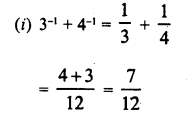Question 3.
Find the values of each of the following :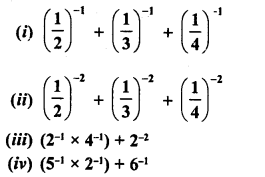Solution: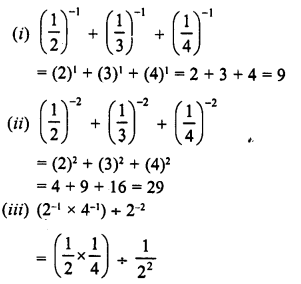Question 4.
Simplify :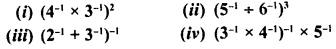Solution: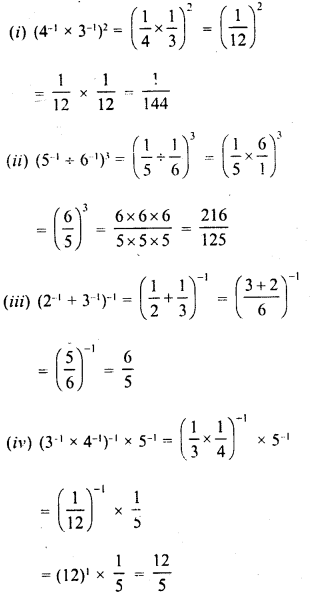Question 5.
Simplify :Solution:Question 6.
By what number should 5-1 be multiplied so that the product may be equal to(-7)-1 ?
Solution:Question 7.
By what number should (12)−1 multiplied so that the product many be equal to (−47)−1
Solution:Question 8.
By what number should (-15)-1 be divided so that the quotient may be equal to (-5)-1 ?
Solution:All Chapter RD Sharma Solutions For Class 8 Maths

—————————————————————————–

All Subject NCERT Exemplar Problems Solutions For Class 8

All Subject NCERT Solutions For Class 8

*************************************************

I think you got complete solutions for this chapter. If You have any queries regarding this chapter, please comment on the below section our subject teacher will answer you. We tried our best to give complete solutions so you got good marks in your exam.

If these solutions have helped you, you can also share rdsharmasolutions.in to your friends.